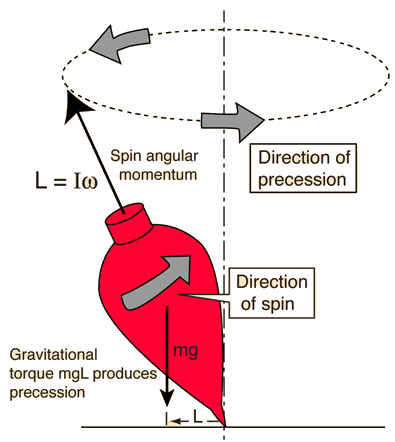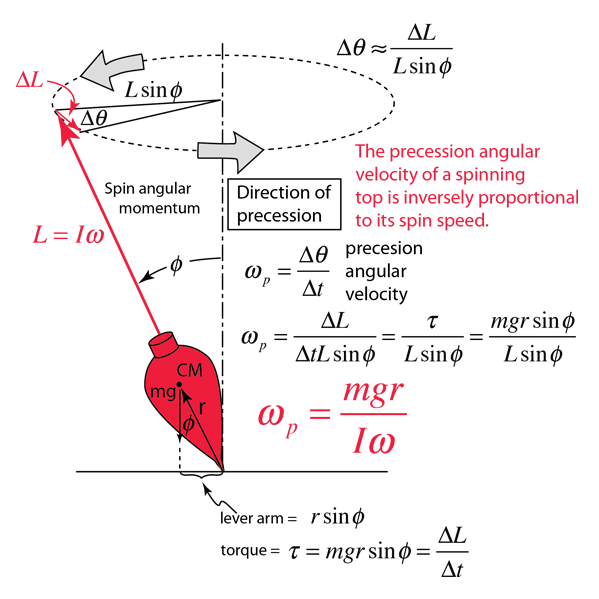# Precession of Spinning TopA rapidly spinning top will precess in a direction determined by the torque exerted by its weight. The precession angular velocity is inversely proportional to the spin angular velocity, so that the precession is faster and more pronounced as the top slows down. The direction of the precession torque can be visualized with the help of the right-hand rule.

Spin a top on a flat surface, and you will see it's top end slowly revolve about the vertical direction, a process called precession. As the spin of the top slows, you will see this precession get faster and faster. It then begins to bob up and down as it precesses, and finally falls over. Showing that the precession speed gets faster as the spin speed gets slower is a classic problem in mechanics. The process is summarized in the illustration below.This process involves a considerable number of physical and mathematical concepts. The angular momentum of the spinning top is given by its moment of inertia times its spin speed but this exercise requires an understanding of it's vector nature. A torque is exerted about an axis through the top's supporting point by the weight of the top acting on its center of mass with a lever arm with respect to that support point. Since torque is equal to the rate of change of angular momentum, this gives a way to relate the torque to the precession process. From the definition of the angle of precession, the rate of change of the precession angle θ can be expressed in terms of the rate of change of angular momentum and hence in terms of the torque.

The expression for precession angular velocity is valid only under the conditions where the spin angular velocity ω is much greater than the precession angular velocity ωP. When the top slows down, the top begins to wobble, an indication that more complicated types of motion are coming into play.

Index

Vector rotation examples

 HyperPhysics***** Mechanics ***** Rotation R Nave
Go Back